# Inserting in a Linked List

## Inserting a new node in a Linked List in C Language:

In this article, I am going to discuss Inserting a new node in a linked list using C Language with Examples. Please read our previous article, where we discussed How to Improve Searching in Linked List using C Language with Examples.

##### How to Insert a new node in a linked list:

Here, we will see how to insert a new node in an existing linked list.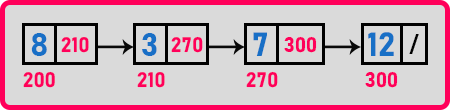In the above Linked List, we want to insert a new node. For insertion, we have to mention at what position we want to insert or at what index we want to insert. Let us give indices to the nodes of the above linked list.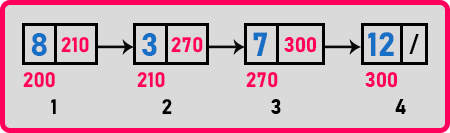So we have given indices. Usually in array, we start index from ‘0’ onwards so that is given by the compiler itself. But here is we can the indices from ‘1’ also or if you want you can change it to ‘0’ onwards. Now let us see what are the places that are available where we can insert a new node.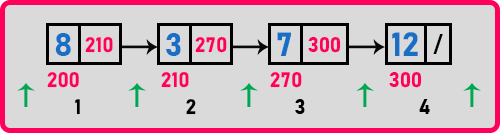Here green arrow represents the places where we can insert a new node.

1. We can insert a new node before the first node.
2. We can insert in between two nodes.
3. We can insert a new node after the last node.

Let us give indices for these positions.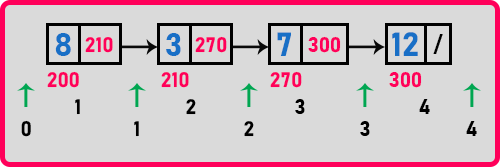So let us say the position before first node as ‘0’ and rest of the positions are ‘0’ onwards. If we say we want to insert at index ‘1’ then it means we want to insert after the first node. If we say at index ‘3’ means after the third node. Here we have to cases:

1. Insert before first.
2. Inserting after given position.

Let us look at the first case inserting before the first.

##### Insertion before first in a Linked List: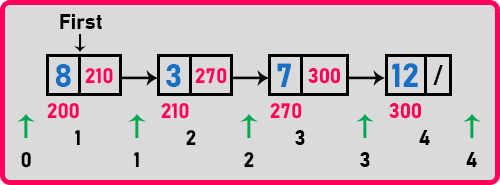Now if we are inserting a new node before first node than we have to move ‘first’ point also because that new node becomes first node otherwise inserting after any node there is no change in ‘first’, simply we can insert a new node at any given index.

First step is to create a new node help of a pointer. Let us say it as ‘t’. Then after creating a new node initialized that node whatever the data, you want to insert. So, let’s say we want to insert ‘4’ Next, we have to modify ‘t->next’ to point where the ‘first’ pointer is pointing. Then next step is to modify pointer ‘first’ to point on this node ‘t’ because it’s getting inserted before the first node. So ‘first’ should point on this.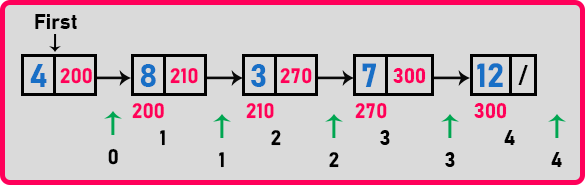So, there are 4 steps involved:

1. Create a node: Node *t = new Node;
2. Insert the data: t->data = x;
3. Make t’s next to point on first: t->next = first;
4. Make the node as first node: first = t;

These are the constant steps so time complex to inserting before first node is O (1). It will take constant time. Now next let us learn how to insert a new node at a given position.

##### Inserting after given position in a Linked List:Let’s say we want to insert at position ‘2’. First, we have to create a new node, so we will take a temporary pointer and create a new node, and initialized it with some data whatever you want to insert.

Next, we have to make ‘t->next’ to point on the next node and make previous nodes next to the point on the t’s node. In this case, t’s next will point on the ‘7’ node and ‘3’ next will point on t’s node. So, these are the two changes we have to make.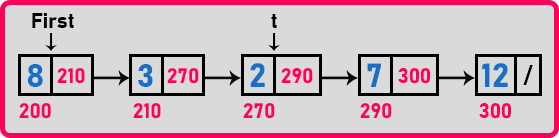So, for modifying ‘7’ we should know the address of that node and to modify ‘2’ next we should have some pointer for accessing this node then only we can access that one or modify that one. For having on previous node, we should bring a pointer from the first node onwards, we cannot directly get a pointer on the previous node.

So, we will create a pointer let say ‘p’ and bring this to that node after which we want to insert a new node. In this case we want ‘p’ at ‘3’.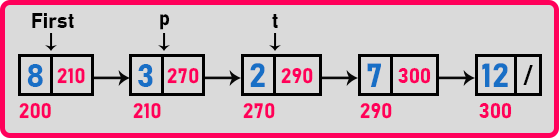For inserting a new node let say at index ‘2’ then we have to move ‘p’ for only 1 time and let we want to insert after index ‘3’ then ‘p’ will move 2 times from the beginning of the linked list. So ‘p’ will move position – 1 time.

The next step is we want the address of the next node to modify the links. Let say here we have inserted a new node after index ‘2’ and ‘p’ is pointing the index ‘1’ then we want the address of next node which is ‘7’ node. So, we will get that by ‘p->next’. Now the last step is ‘p->next’ have to point on ‘t’.

##### Pseudo Code for the above steps:

Node *t = new Node;
t->data = x;
p = first;
for (i = 0; i < pos – 1; i++)
{
p = p->next;
}
t->next = p->next;
p->next = t;

The same procedure or same steps will work on insertion after the last node. Now let us write a function to insert at any position in the linked list.

##### Function to insert a node at any position in a linked list:
```void Insert(int pos, int x)
{
Node *t, *p;
if (pos == 0)
{
t = new Node;
t->data = x;
t->next = first;
first = t;
}
else if (pos > 0)
{
p = first;
for (int i = 0; i < pos - 1 && p; i++)
p = p->next;
if (p)
{
t = new Node;
t->data = x;
t->next = p->next;
p->next = t;
}
}
}
```

Now let us write a full program for insertion a new node in a linked list using C Language.

##### Program for insertion of a new node in a linked list using C Language:
```#include <stdio.h>
#include <stdlib.h>
struct Node
{
int data;
struct Node *next;
}   *first = NULL;

void Create(int A[], int n)
{
int i;
struct Node *t, *last;
first = (struct Node *) malloc(sizeof(struct Node));
first->data = A;
first->next = NULL;
last = first;

for (i = 1; i < n; i++)
{
t = (struct Node *) malloc(sizeof (struct Node));
t->data = A[i];
t->next = NULL;
last->next = t;
last = t;
}
}

int Count(struct Node *p)
{
int l = 0;
while (p)
{
l++;
p = p->next;
}
return l;
}

void Display(struct Node *p)
{
while (p != NULL)
{
printf ("%d ", p->data);
p = p->next;
}
}

void Insert(struct Node *p, int index, int x)
{
struct Node *t;
int i;
if (index < 0 || index > Count(p))
return;
t = (struct Node *) malloc (sizeof (struct Node));
t->data = x;

if (index == 0)
{
t->next = first;
first = t;
}
else
{
for (i = 0; i < index - 1; i++)
p = p->next;
t->next = p->next;
p->next = t;
}
}

int main()
{
int A[] = { 8, 3, 7, 12 };
Create (A, 4);
Insert (first, 2, 2);
Display (first);
return 0;
}
```
###### Output: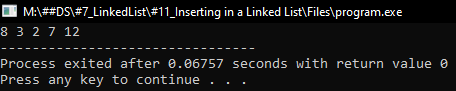In this article, I am going to discuss How to Create a Linked list using Insert using C Language with Examples. Here, in this article, I try to explain How to Insert a new node in a linked list using C Language with Examples and I hope you enjoy this Inserting a new node in a linked list using C Language with Examples article.